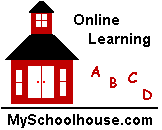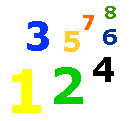# Hundreds Place Value

Place Value Lesson & Worksheet - Kids Math Lessons - My Schoolhouse - Online Learning

Two-digit and three-digit numbers use the tens and hundreds place value.

The numbers 32 and 16 are called "two-digit" numbers.

The numbers 521 and 345 are "three-digit" numbers.

Notice that 521 and 345 have one more digit.

The first digit is in the hundreds place, the second digit is in the tens place and the third digit is in the ones place.

 HUNDREDS TENS ONES 5 2 1 3 4 5

Practice writing three-digit numbers.  Fill in the boxes below.

 THREE DIGIT NUMBER HUNDREDS TENS ONES 241 2 1 466 6 6 542 5 976 125 1 5 360 0 722 7 653 6 5 888 234 3 4 575 5 219 1